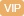| APP | 校园号 | 客服 客服热线：400-863-9889

400-863-9889【考点】函数单调性的性质与判断【专题】函数思想；转化法；函数的性质及应用．
【分析】设列为{an}易a1+a3+…+a2n1=
 (a1+an+1)(n+) 2
=4，a2+4+…a2n=
 (a2+a2n)n 2
=33，利等差列的质可得到
 n+1 n
=
 4 3
，从可得答案．
【解答】解：设该列为{an，依题意，a1+a3+…an1=
 (a1+a2n1)n+1) 2
=4a2+a4+…+a2=
 (a2+a2n)n 2
=3，

【点评】本题查等差列的性质，到
 n+1 n
=
 4 3

0/0